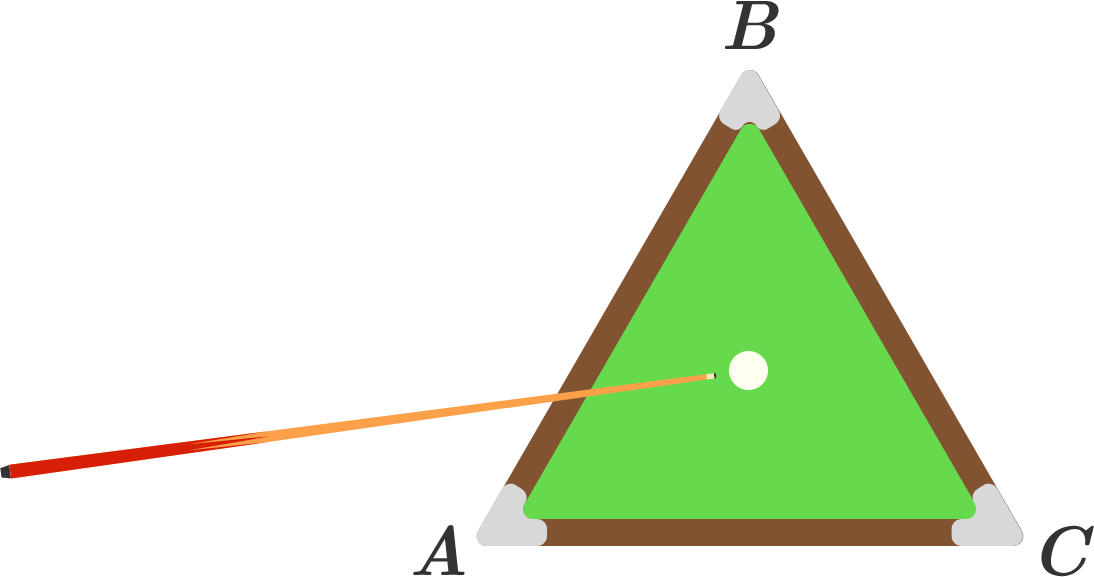# Equilateral Triangle Billiards

Probability Level 4

You are playing pool on a billiard table which is an equilateral triangle $ABC.$ The ball is at the center of the triangle, and you hit it in any direction with uniform probability. What is the expected number of sides the ball hits before hitting side $\overline{AB},$ to 3 decimal places?Details and Assumptions:

• The ball bounces off the sides of the table according to the law of reflection.
• If the first side the ball hits is $\overline{AB},$ then the number of walls the ball hits before hitting $\overline{AB}$ is zero.
• The ball is infinitesimally small.
×